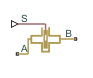# Gate Valve

(To be removed) Hydraulic gate valve

The Hydraulics (Isothermal) library will be removed in a future release. Use the Isothermal Liquid library instead. (since R2020a)

## Library

Flow Control Valves

•## Description

The Gate Valve block models a valve consisting of a round orifice in the valve housing and a flat gate, which moves perpendicular to the orifice axis. The orifice in the gate has the same diameter as the orifice in the housing. As the gate moves, it opens or closes the valve passage (shown as a shaded area in the following illustration).The flow rate through the valve is proportional to the valve opening and to the pressure differential across the valve. The flow rate is determined according to the following equations:

`$q={C}_{D}\cdot A\left(h\right)\sqrt{\frac{2}{\rho }}\frac{\Delta p}{{\left(\Delta {p}^{2}+{p}_{\text{Cr}}^{2}\right)}^{1/4}},$`
`$\Delta p={p}_{\text{A}}-{p}_{\text{B}},$`
`$h={x}_{0}+x$`

where

 q Flow rate p Pressure differential pA, pB Gauge pressures at the block terminals CD Flow discharge coefficient A(h) Instantaneous orifice passage area x0 Initial opening x Gate displacement from initial position h Valve opening D Orifice diameter ρ Fluid density Aleak Closed valve leakage area pcr Minimum pressure for turbulent flow

The minimum pressure for turbulent flow, pcr, is calculated according to the laminar transition specification method:

• By pressure ratio — The transition from laminar to turbulent regime is defined by the following equations:

pcr = (pavg + patm)(1 – Blam)

pavg = (pA + pB)/2

where

 pavg Average pressure between the block terminals patm Atmospheric pressure, 101325 Pa Blam Pressure ratio at the transition between laminar and turbulent regimes (Laminar flow pressure ratio parameter value)
• By Reynolds number — The transition from laminar to turbulent regime is defined by the following equations:

`${p}_{cr}=\frac{\rho }{2}{\left(\frac{{\mathrm{Re}}_{cr}\cdot \nu }{{C}_{D}\cdot {D}_{H}}\right)}^{2}$`
`${D}_{H}=\sqrt{\frac{4A}{\pi }}$`

where

 DH Valve instantaneous hydraulic diameter ν Fluid kinematic viscosity Recr Critical Reynolds number (Critical Reynolds number parameter value)

Connections A and B are hydraulic conserving ports. Connection S is a physical signal port that controls the gate displacement. The block positive direction is from port A to port B. This means that the flow rate is positive if it flows from A to B, and the pressure differential is determined as $\Delta p={p}_{\text{A}}-{p}_{\text{B}},$. Positive signal at the physical signal port `S` opens the valve.

## Basic Assumptions and Limitations

• No inertial effects are taken into account.

## Parameters

Valve orifice diameter

The diameter of the valve orifice. The orifices in the valve housing and in the gate have the same diameter. The default value is `0.01` m.

Initial opening

The initial opening of the valve. The parameter can take both positive and negative values. The default value is `0`.

Flow discharge coefficient

Semi-empirical parameter for valve capacity characterization. Its value depends on the geometrical properties of the orifice, and usually is provided in textbooks or manufacturer data sheets. The default value is `0.65`.

Laminar transition specification

Select how the block transitions between the laminar and turbulent regimes:

• `Pressure ratio` — The transition from laminar to turbulent regime is smooth and depends on the value of the Laminar flow pressure ratio parameter. This method provides better simulation robustness.

• `Reynolds number` — The transition from laminar to turbulent regime is assumed to take place when the Reynolds number reaches the value specified by the Critical Reynolds number parameter.

Laminar flow pressure ratio

Pressure ratio at which the flow transitions between laminar and turbulent regimes. The default value is `0.999`. This parameter is visible only if the Laminar transition specification parameter is set to `Pressure ratio`.

Critical Reynolds number

The maximum Reynolds number for laminar flow. The value of the parameter depends on the orifice geometrical profile. You can find recommendations on the parameter value in hydraulics textbooks. The default value is `10`. This parameter is visible only if the Laminar transition specification parameter is set to `Reynolds number`.

Leakage area

The total area of possible leaks in the completely closed valve. The main purpose of the parameter is to maintain numerical integrity of the circuit by preventing a portion of the system from getting isolated after the valve is completely closed. The parameter value must be greater than 0. The default value is `1e-12` m^2.

## Global Parameters

Parameters determined by the type of working fluid:

• Fluid density

• Fluid kinematic viscosity

Use the Hydraulic Fluid block or the Custom Hydraulic Fluid block to specify the fluid properties.

## Ports

The block has the following ports:

`A`

Hydraulic conserving port associated with the valve inlet.

`B`

Hydraulic conserving port associated with the valve outlet.

`S`

Physical signal port that controls the gate displacement. The signal applied to this port is treated as translational motion, in meters.

## Version History

Introduced in R2011b

collapse all

### R2023a: To be removed

The Hydraulics (Isothermal) library will be removed in a future release. Use the Isothermal Liquid library instead.